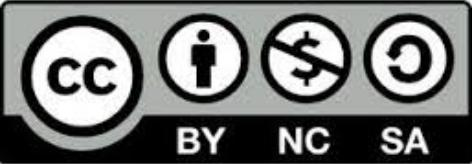Cite as: Alfonso Garmendia (2016) R for life sciences. Chapter 4: Hypothesis testing. http://personales.upv.es/algarsal/Documentation/Garmendia-R-Tutorial-04_Tests.html

available in PDF and EPUBThis work is licensed under a Creative Commons Attribution-NonCommercial-ShareAlike 4.0 International License.

Written in Rmarkdown, using Rstudio and pandoc.

# Hypothesis testing

There is a lot of information available about all the possible tests. Too much for a single course. But there are a few important things to learn:

• How to choose the right test for your hypothesis and data.

• How to look how to perform it.

Therefore, what you have here is:

• A table to help choosing the right test

• A compilation of web places where start looking how to perform the basic (and not so basics) tests.

## Which test should I do?

To choose the right test depending on the number and nature of dependent and independent variables, you can use this table, also available in PDF or EPUB.

## Population mean and proportion

A good tutorial for one and two tails tests for means and proportions is:

http://www.r-tutor.com/elementary-statistics/hypothesis-testing

### mean comparison

For the comparison of means between samples:

http://www.r-tutor.com/elementary-statistics/inference-about-two-populations

Also, look at the anova tests and TukeyHSD posthoc comparisons to compare means in linear models.

## Linear models

This is a good introduction to linear models and generalized linear models:

https://www.r-bloggers.com/an-intro-to-models-and-generalized-linear-models-in-r/

### Linear Regression

Very well explained here:

http://tutorials.iq.harvard.edu/R/Rstatistics/Rstatistics.html

### Anova

A good tutorial on anova:

http://www.statmethods.net/stats/anova.html

Anova is a type of linear model. It is possible to make an anova using lm(), but then it will be a “type 3” anova. The command aov() makes by default a “type 1” anova. Also, a difference of aov() from lm () is in the way print, summary and so on handle the fit: this is expressed in the traditional language of the analysis of variance rather than that of linear models.

If using anova(lm()), you should get the same results than with aov().

Type 1 or Type 3 sums of squares will differ when the correlation between your explanatory variables is not exactly 0 (it is only important if there is more than one). When they are correlated, some SS are unique to one predictor and some to the other, but some SS could be attributed to either or both. type 1 SS approach is for the analyst to use their judgment and assign the overlapping SS to the first of the variables. The other variable goes into the model second and gets the SS that looks like a cookie with a bite taken out of it.

Alternatively, you could do this twice with each going in first, and report the F change test for both predictors. In this way, neither variable gets the SS due to the overlap. This approach uses Type 3 SS.

Type 3 SS approach is held in low regard, besides it is the default anova in SPSS and other statistical programs.

Type 1: SS(A) and SS(B|A)

Type 3: SS(A|B) and SS(B|A)

## Tests of normality

Normality assumptions are not always so restrictive as people thinks. For linear models the only normality assumption needed is the normality of residuals. It is very well explained at:

https://www.r-bloggers.com/predictors-responses-and-residuals-what-really-needs-to-be-normally-distributed/

For the shapiro.test() it is interesting to see the example(shapiro.test). H0 is normality and H1 is non-normal. For further interpretation: http://emilkirkegaard.dk/en/?p=4452

Remember the Central Limit Theorem that says that if the errors are not normal then the distribution of the coefficients will approach normality as the sample size increases. The more important question is are the residuals “normal enough”? for which there is not a definitive test (experience and plots help). plot(lm()) to check assumptions.

But this all depends on another assumption, that the data is at least interval data. If you do not believe on the at least theoretical normality of the residuals, you can rather use non-parametric analyses. or generalized linear models glm().

## Non-parametric analyses

For groups comparisons:

http://www.statmethods.net/stats/nonparametric.html

## Multivariate analyses

The Little book of R for Multivariate Analyses has a nice explanation for Principal components and Discriminant analyses. But is important to load the data first.

### Correspondence

other with same data: http://factominer.free.fr/classical-methods/correspondence-analys

### Classification analyses

For a complete revision of methods: http://www.sthda.com/english/wiki/cluster-analysis-in-r-unsupervised-machine-learning

# Exercises

1. For the data InsectSpray, make a table for the number of insects for each spray with the mean, median and standard error.
2. Print a box-plot to see the differences of counts between sprays.
3. Test for differences between sprays performing Student’s t-tests1
4. Test for differences between sprays using anova2 and Tukey posthoc comparison3.
5. Test for differences between sprays using non-parametric Kruskal-Wallis rank sum test4.
6. Transform count data using sqrt(counts) and redo the anova and tukey posthoc comparison
7. Test for normality of residuals for the two performed anova analyses of points 4 and 6 using shapiro.test() and plotting the anova to see the qqplots and compare them.
8. ¿Which analysis is the adequate in this case? Why?
9. ¿Are there differences between the results from the different analyses? ¿Which ones?
10. Plot a definitive box-plot with letters indicating significant differences between sprays. Same letter means that there is not a significant difference.

1. t.test(): for means comparison

2. aov()

3. TukeyHSD(): Tukey’s ‘Honest Significant Difference’ method

4. kruskal.test()